# Calibration

Let us illustrate the previous calculation for a sphere receiving uniform energy deposition at time zero. The time sequence for the pressure in a sphere in which thermal energy is deposited at time zero is shown here.

The computer code that generated these figures is written in C and is listed here

Thermal energy deposition Wo = 1 [J/cm3] = 106 [J/m3].
Peak pressure = ΓWo =(0.12)(106 [J/m3]) = 1.2x105 [J/m3] = 1.2x105 [Pa].
(left)Map of P(x,z) [J/m3]. (right)Line graph of P(x)@z=0, and P(z)@x=0.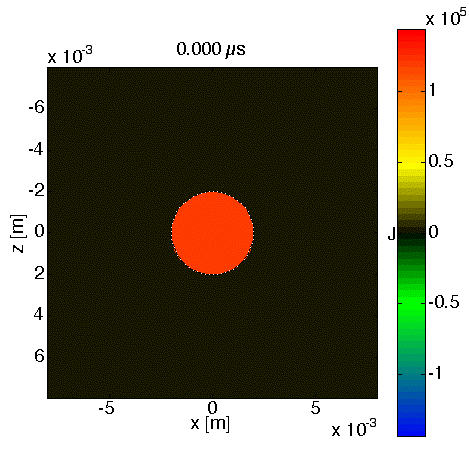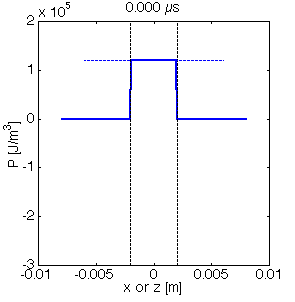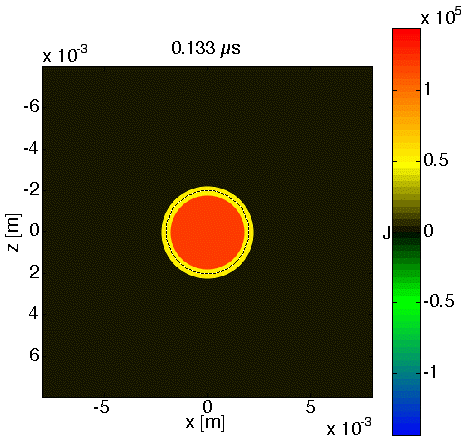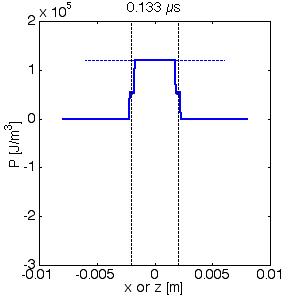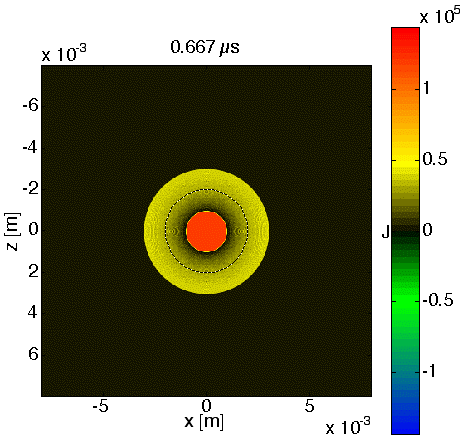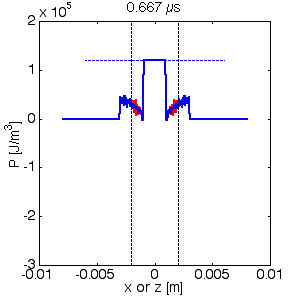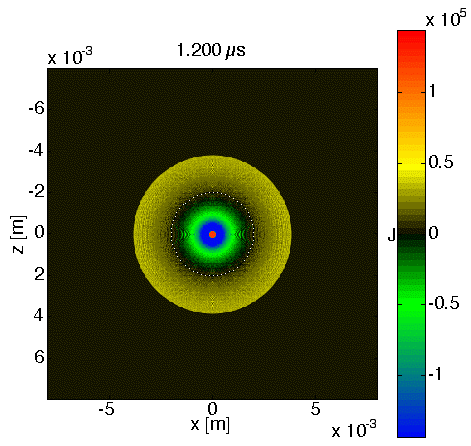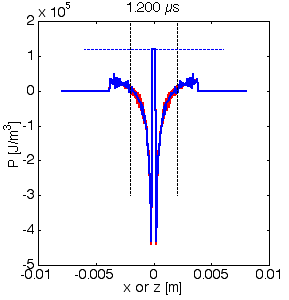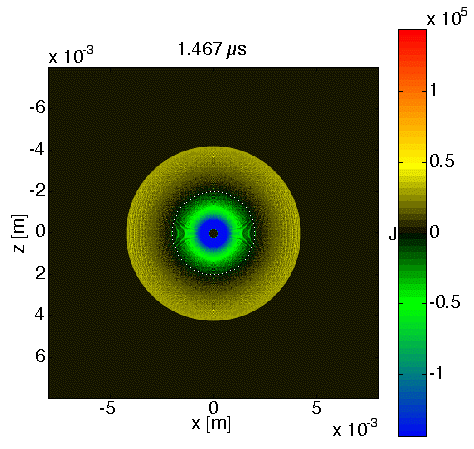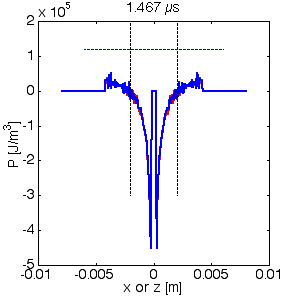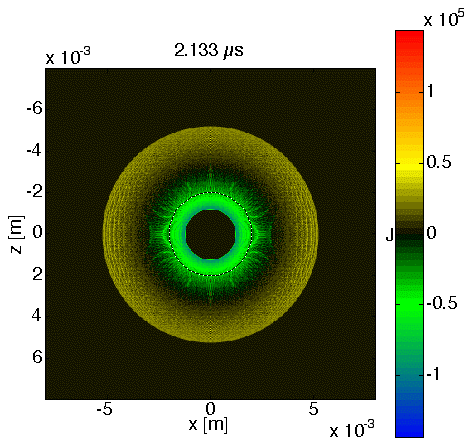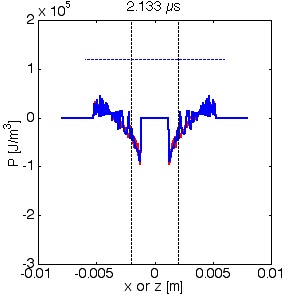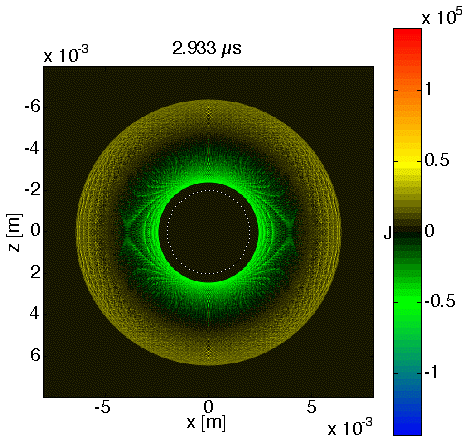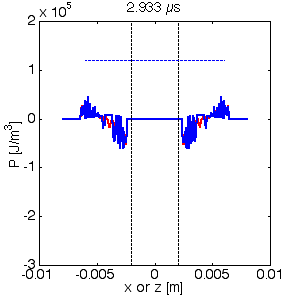The central region of the sphere maintains a constant pressure of 0.12 GPa while a negative pressure release wave moves in toward the center from the surface of the sphere. As this release wave approaches the center, the negative pressure is concentrated. About half way toward the center, it overcomes the positive pressure and yields a net negative pressure. When it reaches the center, an impulse of negative stress occurs at the center. Thereafter, the pressure at the center becomes equal to zero, and the region of zero pressure grows outward. Meanwhile, a positive compressive pressure wave moves outward from the sphere surface, followed by a negative tensile pressure wave.

This computation still suffers from some computational noise. The answer should be radially symmetric. Work in progress :-)